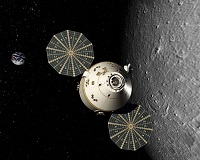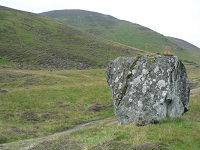﻿ Mechanics Map - Newton's First Law

# Newton's First Law

Newton's first law states that: "A body at rest will remain at rest unless acted on by an unbalanced force. A body in motion continues in motion with the same speed and in the same direction unless acted upon by an unbalanced force."

This law, also sometimes called the "law of inertia", means that bodies maintain their current velocity unless a force is applied to change that velocity. If an object is at rest with zero velocity it will remain at rest until some force begins to change that velocity, and if an object is moving at a set speed and in a set direction it will remain at that same velocity until some force begins to change that velocity.In the absence of friction in space, this space capsule will maintain it's current velocity until some outside force causes that velocity to change. Public Domain image by NASA.This rock is at rest with zero velocity and will remain at rest until a net force causes the rock to move. The net force on the rock is the sum of any force pushing the rock and the friction force of the ground on the rock opposing that force. Image by Liz Gray CC-BY-SA 2.0.

## Net Forces:

It is important to note that the net force is what will cause a change in velocity. The net force is the sum of all forces acting on the body. For example, we can imagine gently pushing on the rock in the figure above and observing that the rock does not move. This is because we will have a friction force equal in magnitude and opposite in direction opposing our gentle pushing force. The sum of these two forces will be equal to zero, therefore the net force is zero and the change in velocity is zero.

## Rotational Motion:In the absence of friction, this spinning top would continue to spin forever, but the small frictional moment exerted at the point of contact between the top and the ground will slow the tops spinning over time. Image by Carrotmadman6 CC-BY-2.0.

Newton's first law also applies to moments and rotational velocities. A body will maintain it's current rotational velocity until a net moment is exerted to change that rotational velocity. This can be seen in things like toy tops, flywheels, stationary bikes, and other objects that will continue spinning once started until brakes or friction stop them.